# Compensating camera MTF measurements for chart and sensor MTF

Current Documentation
View previous documentation
View legacy documentation
All documentation versions

 Summary— Starting with Imatest 5.1 MTF measurements can be compensated for measured chart quality. Chart MTF compensation can double the megapixel suitability of a test chart. Results taken with different test charts will be more consistent. An approximate lens MTF calculation (independent of chart MTF compensation) has been added. It is based on a geometrical model of sensor MTF, which is used because reliable sensor MTF measurements are difficult to obtain. It’s not perfect, but it is good enough for many measurements. A Chart Quality Calculator lets you determine the suitability of a test chart (based on the camera’s Vertical Field of View, vertical pixel count, and modeled MTF) for a specific application. The Chart MTF Suitability plot makes this particularly convenient. Test chart suitability for MTF measurements has chart MTF suitability displays for several media types. Each chart is based on an MTF compensation file (which models measurements of the chart). All you need to characterize the megapixel suitability of a chart is the vertical Field of View (VFoV) and number of vertical pixels in the camera image sensor.

### Introduction

Imatest MTF measurements are affected by both chart and camera quality. Since its inception, Imatest has recommended test charts that are large enough to minimize the effects of the chart on camera MTF measurements. Although we have some descriptions of chart quality on the Chart Quality Index, Reflective charts, and Transmissive charts quality pages, these pages don’t have enough detail to determine the precise effect of chart MTF on camera MTF measurements, which is particularly important in situations where geometric constraints (test equipment and lab size, etc.) make it difficult to meet the chart recommendations.

Convolution and deconvolution –  The response of a linear system can be calculated by convolving its components. Convolution is a complex operation— as its name implies— but when response is converted into frequency domain (by means of a Fourier transform), it becomes simple multiplication. The measured response (Modulation Transfer Function = MTF) of an imaging system is the product of its elements:

$$MTF_{system-measured} = MTF_{chart-projected} \times MTF_{lens} \times MTF_{sensor} \times MTF_{image-processing}$$

MTFchart-projected (the chart MTF projected on the image sensor) is not really a part of the imaging system, so we’ve dealt with it by recommending that charts be used in conditions (low magnification) where MTFchart-projected stays close to 1 in the frequency range of interest. Since our recommendations have been conservative the results have been “good enough” in most instances.

Starting in Imatest 5.1 the effects of the chart can be reduced by dividing the measured MTF by MTFchart-projected, which is equivalent to deconvolution in the spatial domain. We can also calculate the approximate lens MTF by dividing measured MTF by a geometrical model of MTFsensor, which we use because (A) reliable measurements of MTFsensor are difficult to obtain, and (B) results are closer to lens MTF than you can get without the correction.

Chart-compensated MTF model   Chart MTF is measured in units of Cycles per Object mm from a printed edge (appropriately slanted for the measurement), then fit to a simple equation that fits a wide range of chart MTF measurements.

$$\displaystyle MTF_{chart} (f) \cong e^{-a_1 f – (a_2 f)^2}$$;      f in units of Cycles/Object mm.

When the chart is imaged (projected) on the sensor, the projected chart MTF is calculated by transforming the chart frequency units to the native sensor units (Cycles/Pixel) using

$$f(\text{C/Obj mm}) = f(C/P) \times \text{magnification} \times \text{pixels/mm}$$

$$MTF_{chart-projected}(f) = MTF_{chart}(f(C/P) \times \text{magnification} \times \text{pixels/mm}) = MTF_{div}(f)$$

In Imatest sharpness modules, pixels/mm is typically derived from the user-entered value of pixel pitch (in μm), where pixels/mm = 1000 / (pixel pitch (μm)). Magnification is either entered in the settings window or derived from geometrical factors (like the bar-to-bar spacing in SFRplus or the vertical registration mark spacing in eSFR ISO). We use MTFdiv(f) and MTFchart-projected(f) interchangeably because the measured camera MTF (MTFmeasured) is divided by MTFdiv to obtain the compensated (more accurate) measurement.

If MTFdiv at the Nyquist frequency (fNyq = 0.5 C/P) is lower than 0.9 (90%), measurement accuracy can be improved by dividing the measured MTF by MTFdiv. If MTFdiv(fNyq) is greater than 0.9, there is very little improvement.

$$\displaystyle MTF_{chart-compensated}(f) =\frac{ MTF_{measured}(f)}{MTF_{div}(f)}$$

The actual calculation (details below) is performed with files that contain parametric fits to the measured data for the specific media type and printing technology. Applying chart compensation will improve the consistency of MTF measurements taken on different test charts.

Lens MTF measurements –  Imatest traditionally measures the MTF of the entire system, lens + sensor + image processing, but a number of customers are interested in measuring the MTF of the lens alone. To do so, the effects of the sensor and image processing must be removed. It’s easy to minimize image processing by converting files from raw  using dcraw (for commercial files) or Generalized Read Raw (for binary files), but removing the effects of the image sensor is more difficult.  Although exact measurements of sensor MTF are difficult to come by (as of early 2018) we can get a reasonable approximation for many situations (visible light, moderate pixel size) using a simple theoretical equation for geometrical sensor MTF:

$$\displaystyle MTF_{sensor} =\frac{ \sin \frac{\pi f}{2 f_{Nyq}}}{ \frac{\pi f}{2 f_{Nyq}}} = \text{sinc} \frac{\pi f}{2 f_{Nyq}}$$  where  fNyq = Nyquist frequency = 1/(2 pixel pitch).

For this equation to be valid the sensor must not have an anti-aliasing filter (OLPF) and a raw image must be used (converted with dcraw or Read Raw). JPEG images from cameras inevitably have signal processing (sharpening and noise reduction) that throws off the results. This approximation is reasonably good for visible light, but poorer for Near Infrared (NIR), where electron diffusion in the silicon degrades the MTF.

### Chart-compensated MTF calculation

Starting with Imatest 5.1 you can compensate MTF measurements for chart sharpness. Compensation is available in all slanted-edge modules (SFR, SFRplus, eSFR ISO, SFRreg, and Checkerboard), and for Star, Random (Dead Leaves, Spilled coins, etc.), and Log F-Contrast. Applying chart compensation requires care.

#### Summary of operations

• Read the image, then press the  Chart MTF compensation  button in the settings window.
• Check the  Apply chart MTF compensation  checkbox,
• Read a file that contains a model of the chart MTF (each specific chart medium has a model).
• Enter the sensor pixel spacing (typically in microns) and either the magnification or the parameters that can be used to calculate magnification (for example, the bar-to-bar spacing in SFRplus).
• Run the analysis as usual. The settings will be saved for the individual module.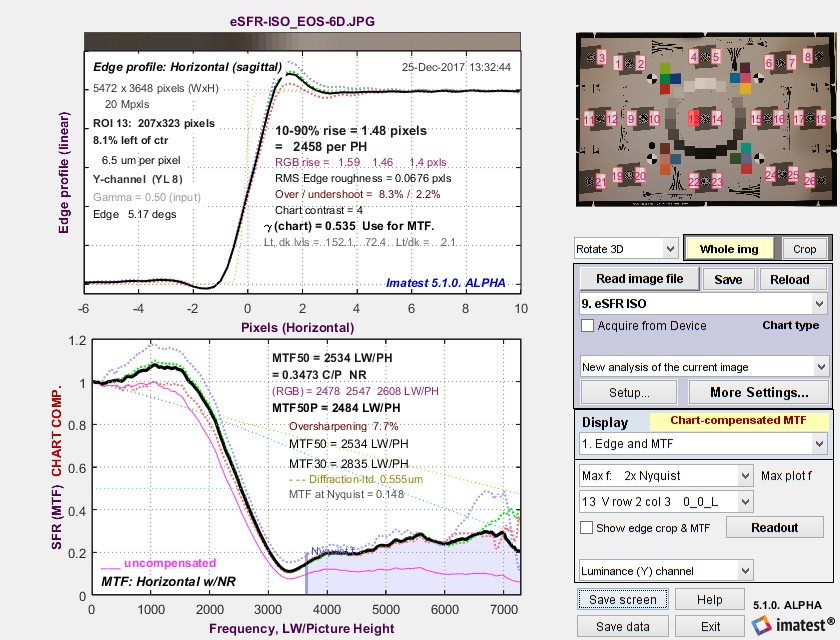Chart-compensated Edge MTF plot.  Uncompensated MTF shown in magenta ____.

#### Chart MTF compensation files

Click on a link in the table  to download the chart compensation file for the indicated media type. Save the file to any convenient location. (We recommend the Imatest Resources folder, which can be located by pressing  Open resources folder  in the Chart and sensor MTF compensation or Options II menu.) Although the files contain several lines, only the numbers in the first line (the first line of Key values in the table below) are used for chart compensation. The remaining lines contain annotations (the file used for the calculation, the chart MTF50, etc.) Some of the data is relatively old: we will be taking new measurements.

 Media Link Key values* B&W Photographic film (LVT process) BW_LVT_film_MTF_compensation.csv -0.00942, -0.04480 MTF50 = 20.8 C/Obj mm Color Photographic film (LVT process) Color_LVT_film_MTF_compensation.csv -0.00968, 0.04781 MTF50 = 18.2 C/Obj mm Inkjet: Displaytrans transparency DisplayTrans_4-1_compensation.csv 0.02733, 0.20737 MTF50 = 3.56 C/Obj mm Inkjet: Epson Matte Enhanced_Matte_precision_MTF_compensation.csv 0.01998, 0.24561 MTF50 = 3.22 C/Obj mm Inkjet: Epson Hot Press Bright Epson_Hot_Press_Bright_BW_MTF_compensation.csv 0.08608, 0.13545 MTF50 = 3.0 C/Obj mm B&W Photographic paper Photo_Paper_MTF_compensation.csv 0.10603, 0.00001 MTF50 6.44 C/Obj mm

*The first key value line contains all information required for the Chart Compensation file. The second line (MTF50 in Cycles/Object mm) is included for reference— for conveniently comparing chart sharpness.

We plan to supply MTF compensation files in Imatest builds. They will also be available for downloading in the Reflective charts and Transmissive charts quality pages.

You can create an MTF compensation file using the technique described below in Appendix: Chart MTF measurement. This file should be saved with a descriptive name to any convenient location (though we recommend the Resources folder).

#### Applying MTF compensation

• Read the image in one of the sharpness modules that supports chart compensation.
• In the Settings window (the  More settings  window in Rescharts slanted-edge modules), Enter the sensor pixel spacing (typically in microns) and either the magnification or the parameters that can be used to calculate magnification (for example, the bar-to-bar spacing in SFRplus or the vertical registration mark spacing in eSFR ISO).
• Press the  Chart MTF compensation  button ( MTF compensation  in Rescharts slanted-edge modules). This opens the window shown on the right.
• If a file has been read (and stored) a plot like the one on the right will be displayed. If not, press the  Read the compensation file  button and select the appropriate compensation file for the test chart media.
• Check the  Apply chart MTF compensation   checkbox.
• Click OK.
• Run the analysis as usual. The settings will be saved for the individual module.

Chart compensation will increase MTF. It will be indicated in several places.

• in plot titles (in many cases),
• in the y-label of MTF plots, which will typically change from MTF to MTF CHART COMPENSATED (or MTF CHART_COMP. if space is tight),
• In Rescharts, Chart or lens MTF comp. will appear on the right of the window.

#### Chart measurements for calculating magnification

SFRplus:  Bar-to-bar height (top of top bar to bottom of bottom bar) in cm.

eSFR ISO:  Registration mark vertical spacing in cm.

Checkerboard:  Square height (not shown) in cm.

For these three modules the chart should be measured carefully. The measurement is entered on the right of the Settings window.

Magnification must be entered directly for all other modules.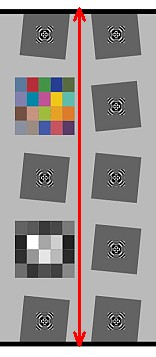SFRplus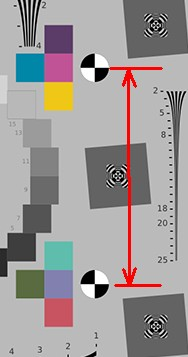eSFR ISO

 Things to check carefully  Using chart compensation is somewhat more error-prone than not using it. You need to check settings carefully. Make sure you enter the correct compensation file for your media (inkjet, LVT file, etc.) you enter the correct pixel pitch (spacing) for your image sensor you enter the magnification or a measurement used to calculate the magnification (Bar-to-bar spacing in SFRplus, Registration mark spacing in eSFR ISO, or Square height in Checkerboard) you have turned on the correct compensation. Chart and Sensor MTF compensation are completely independent, even though the share the same window and perform similar functions. MTF compensation increases measured MTF values at high spatial frequencies. This often means that noise as well as the signal will be boosted. This is very visible in the above image, taken with a Canon EOS-6D DSLR, which has an anti-aliasing filter that removes most response above Nyquist (~3600 LW/PH). What is left is primarily noise.

#### Effects of MTF compensation

Here is a typical example, run in Rescharts for a Star chart image. Indicators appear in the y-label, title, and on the middle-right of the Rescharts window. The compensation file name (media type) does not yet appear. Two additional curves appear on the plot. The original MTF without compensation appears as a Magenta dotted line ………..The projected MTF of the chart (MTFchart-projected ) is a cyan dotted line ………..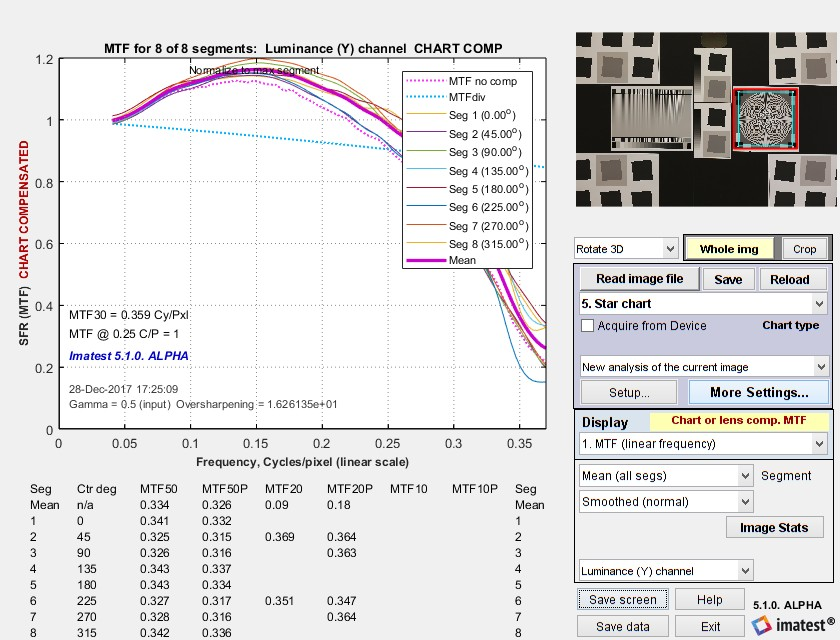Chart MTF-compensated results for Star chart

There are a few things to watch out for when doing these measurements.

To prevent excessive noise boost MTFchart-projected (the cyan dotted line ……….) must not drop too low (to 0.3) in the frequency range of interest.

### Sensor MTF compensation for approximate Lens MTF measurements

We receive frequent requests from customers who want to measure lens MTF, and it’s always disappointing to tell them Imatest measures system MTF, which includes the effects of the image sensor, and not lens MTF. While sensor MTF can be removed by deconvolution, i.e., the same division process (in frequency domain) used to compensate for the chart MTF, reliable sensor MTF data is difficult to obtain. But even without exact sensor MTF data Imatest can do a partial (approximate) correction for the sensor MTF.

Sensor MTF actually consists of two or three components.

1. a geometrical component derived from the pixel dimensions. Its equation is
$$\displaystyle MTF_{sensor} = \frac{\sin \bigl( \pi f / (2 f_{Nyq})\bigr) }{ \pi f / (2 f_{Nyq})} = \text{sinc} \bigl( \frac{\pi f}{2 f_{Nyq}}\bigr)$$ where  fNyq = Nyquist frequency = 1/(2 pixel pitch).
Note that this equation has a null at f = 2 fNyq, i.e., twice the Nyquist frequency. Sensor response at fNyq is 2/π = 0.6366.

2. an electrical component caused by diffusion of light inside the silicon, resulting in crosstalk, which degrades MTF. This degradation is difficult to measure. Crosstalk is affected by sensor technology and is worse at longer (Near Infrared = NIR) wavelengths and for very small pixels. It is discussed in the Adimec  page on sensor MTF.

3. (not in all cameras) an anti-aliasing (AA) filter, sometimes called an Optical Low Pass Filter (OLPF), frequently found in older DSLR or mirrorless cameras with interchangeable lenses. An AA filter is typically a plastic layer mounted in front of the sensor that reduces aliasing (visible as stair-stepping or Moiré) by blurring the image. Aliasing is caused by strong response above fNyq with sharp lenses. Nikon has a nice article comparing the performance of similar cameras with and without AA filters. AA filters are true wildcards in their effect on sensor MTF response— we have never seen a response curve or equation for AA filter response. All we can say is that they significantly reduce response above and have some effect on response below fNyq.

Imatest can correct for the geometrical component of lens MTF in cameras without anti-aliasing filters. Before you apply this correction, you should find out if your camera has one. Most DSLR and mirrorless interchangeable cameras made before 2015 have them. Since 2015 a number of cameras have small enough pixels (sufficient megapixels) to dispense with them with minimal risk of Moiré. Since removing the AA filter significantly improves camera sharpness (beyond the simple megapixel increase) it may be mentioned in the camera specifications and in reviews. For example, see Wired’s review of the Panasonic GX85

To apply the lens MTF correction, check the Apply sensor MTF compensation checkbox in the compensation window, shown above.

 These results should not be represented as true lens MTF. At best it’s approximate— closer to true lens MTF than measurements made without the correction, but definitely not all the way there. Sensor MTF compensation should only be applied to cameras without anti-aliasing filters.

A recent reference to sensor MTF is Interferometric Measurement of Sensor MTF and Crosstalk (2017) by T. Georgiev, et. al. of Qualcomm, presented at the Electronic Imaging 2017 conference. They used a Ximea MQ013RG-E2 sensor, which has 5.3μm pixels.

Adimec has a page, CCD vs. CMOS – Modulation Transfer Function (MTF) in NIR, that explains why Sensor MTF is generally worse than the theoretical sinc(πf/(2 fNyq)) model, especially for small pixels and Near Infrared (NIR) wavelengths (> 700μm). It has a plot of  MTF at the Nyquist frequency vs. wavelength for two Sony sensors with relatively large pixels, the ICX674 (4.54μm pixels) and IMX174 (5.86μm pixels). MTF is relatively constant and close to the theoretical value of 0.6366 below 700 nm. The method used to obtain this plot is not stated.

#### Appendix. Chart MTF measurement

Most users won’t be measuring chart quality themselves. They’ll use the measurement results in Chart MTF compensation files provided by Imatest in the above links. Those users can skip this section.

Chart MTF is measured from images of slanted-edges on various media (inkjet paper, photographic paper, B&W or color film, or Chrome on Glass) at the same contrast (usually 4:1 or 10:1) used for testing lenses. These media are both transmissive and reflective and have a wide range of sharpness.

1. Photograph the chart at the appropriate magnification, making sure the magnification is accurately measured and recorded (it can be included in the file name). The magnification should be high enough so the measured MTF is limited by the chart, not by the camera. Measured chart MTF50 should be under 0.1 cycles/pixel to minimize the effects of image processing on the results. This generally requires a high quality prime macro lens. We are currently using a Sony 24-megapixel APS-C camera with a Canon 60mm f/2.8 macro lens (with an adapter). The Canon macro has manual operation, which enables us to maintain a constant magnification (0.5 for inkjet charts; 1 for photographic charts, which are generally sharper). This setup isn’t quite up to measuring Chrome-on-Glass (CoG) charts.

2. Run the image in SFR with the following settings:
MTF plot units = Cycles/Object mm.
Pixel size (3.88 um/pixel for the Sony Alpha A6000, etc.)
Magnification (typically ≤1).
ROI detection & plot extra smoothing should be checked.
Gamma should be set correctly (1 for raw files; ~0.5 for standard color space files).
Max MTF plot Freq should be set ≤ 0.5x Nyquist (may vary) to obtain a good plots. (This setting doesn’t affect the results).
Chart MTF compensation (to be used later) should be unchecked.
In the ROI Options window (accessible from the Imatest main window) SFR ROI filtering should be set to None.
The region should be large enough so that edge roughness (which can be a problem with inkjet charts) does not cause excessive MTF variation. ROI size for inkjet charts can be 600×1000 pixels or larger at 0.5x magnification (which is why lower magnification is advantageous).

3.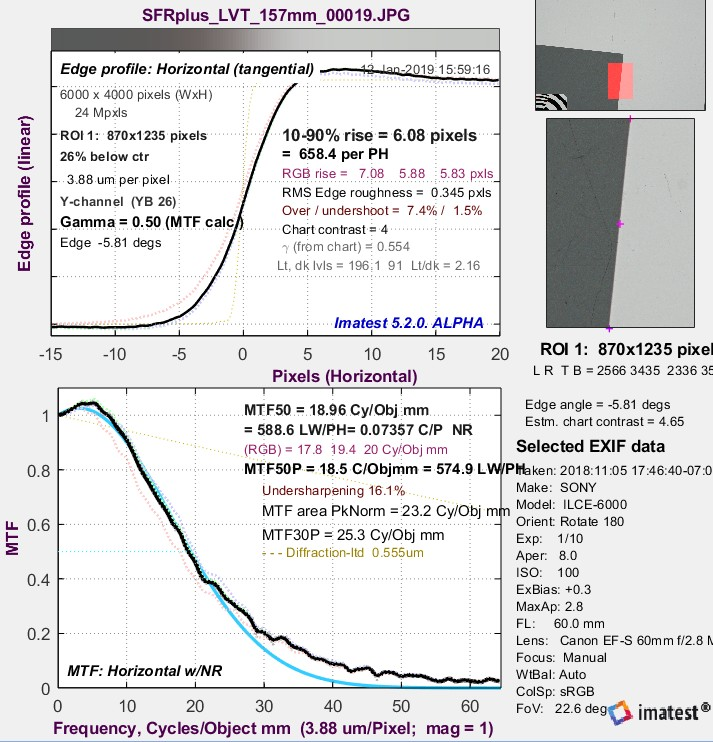Chart MTF for LVT color film chart. Chemical sharpening from dilute film developer causes the response boost.

If the settings are correct, Imatest will calculate a fit to the equation,
$$MTF_{chart} (f) = e^{-a_1 f – (a_2 f)^2}$$
where f has units of cycles/object mm (C/Obj mm).

This equation fits a variety of curve shapes, and can handle response bumps, as shown as a cyan line ____ on the right. An optimizer is used to match the equation to the data for f ≤ MTF30, i.e., only up to the first spatial frequency where MTF is drops below 0.3 (30%).

a1 and a2 are stored in a file, along with metadata (information on how the image was analyzed).

4. The results will be displayed in the command window and copied to the clipboard (for the last ROI-only). Here is a sample of command window text for this image.

— MTF coefficients x for deconvolution (will be copied to clipboard)—

-0.01664, 0.05207, Ver-R
-0.01670, -0.04956, Hor-T
end_data
MTF50{Ver-R), measure:, 19.06, model:, 19.35, C/Obj mm
MTF50{Hor-T), measure:, 20.34, model:, 20.54, C/Obj mm
file, C:\imatest\Data\SFR\Misc\Chart_compensation\Sony download\Uncertain orientation\SFRplus_LVT_157mm_00019.JPG
date_run, 12-Jan-2019 15:59:44
date_mod, 2018-11-05_17-46-40, (closest to capture)
Magnification, 1
aperture 8.0
um_per_pixel, 3.88
Equation, MTF = exp(-x(1)*f-(x(2)*f).^2); f in cycles/object mm

The lines shown in blue are saved to a file, which should have a txt or CSV extension. (If the text isn’t in the clipboard, you can select and copy it.) Only the first line (-0.00968, 0.04781) contains data. The rest consists of comments that you can use to verify that you have the correct settings.

5. For measuring camera MTF  Imatest calculates the chart MTF projected on the image sensor, MTFchart_projected , expressed in the sensor’s native units of cycles/pixel (C/P). Since the spatial frequency of an image projected on the sensor is the spatial frequency on the chart (object) divided by the magnification,

$$MTF_{chart-projected}(f) = MTF_{chart}(f(C/P) \times \text{magnification} \times \text{pixels/mm})$$

Pixels/mm is derived from the pixel pitch (μm per pixel), which is entered in the settings window.

$$\text{pixels/mm} = 1000 / \text{pixel pitch (um/pixel)}$$

The equation for the compensated MTF is

$$\displaystyle MTF_{chart-compensated} = \frac{MTF_{measured}}{\text{max}(MTF_{chart-projected}, 0.3)}$$

MTFchart-projected is also called MTFdiv because it is used to divide MTFmeasured. The minimum value of the denominator must be greater than 0.3 because smaller values are unreliable and can cause an excessive noise boost. The frequency where projected chart MTF drops to 0.3 should be outside the range of interest for the measurement— above the Nyquist frequency fNyq .

### Do you have the latest Imatest Master version?

If you do not already have Imatest Master, purchase or try a free trial of Imatest Master version 5.1 to use the MTF Compensation feature.